• +91 9971497814
• info@interviewmaterial.com

# RD Chapter 13- Complex Numbers Ex-13.4 Interview Questions Answers

### Related Subjects

Question 1 :

Find the modulus and arguments of the following complex numbers and henceexpress each of them in the polar form:

(i) 1 + i

(ii) √3 + i

(iii) 1 – i

(iv) (1 – i) / (1 + i)

(v) 1/(1 + i)

(vi) (1 + 2i) / (1 – 3i)

(vii) sin 120o – i cos 120o

(viii) -16 / (1 + i√3)

We know that the polarform of a complex number Z = x + iy is given by Z = |Z| (cos θ + i sin θ)

Where,

|Z| = modulus ofcomplex number = √(x2 +y2)

θ = arg (z) = argumentof complex number = tan-1 (|y| / |x|)

(i) 1 + i

Given: Z = 1 + i

So now,

|Z| = √(x2 + y2)

= √(12 + 12)

= √(1 + 1)

= √2

θ = tan-1 (|y|/ |x|)

= tan-1 (1/ 1)

= tan-1 1

Since x > 0, y >0 complex number lies in 1st quadrant and the value of θ is 00≤θ≤900.

θ = π/4

Z = √2 (cos (π/4) + i sin (π/4))

Polar form of (1 + i)is √2 (cos (π/4) + i sin(π/4))

(ii) √3 + i

Given: Z = √3 + i

So now,

|Z| = √(x2 + y2)

= √((√3)2 + 12)

= √(3 + 1)

= √4

= 2

θ = tan-1 (|y|/ |x|)

= tan-1 (1/ √3)

Since x > 0, y >0 complex number lies in 1st quadrant and the value of θ is 00≤θ≤900.

θ = π/6

Z = 2 (cos (π/6) + isin (π/6))

Polar form of (√3 +i) is 2 (cos (π/6) + i sin (π/6))

(iii) 1 – i

Given: Z = 1 – i

So now,

|Z| = √(x2 + y2)

= √(12 + (-1)2)

= √(1 + 1)

= √2

θ = tan-1 (|y|/ |x|)

= tan-1 (1/ 1)

= tan-1 1

Since x > 0, y <0 complex number lies in 4th quadrant and the value of θ is -900≤θ≤00.

θ = -π/4

Z = √2 (cos (-π/4) + i sin (-π/4))

= √2 (cos (π/4) – i sin (π/4))

Polar form of (1 – i)is √2 (cos (π/4) – i sin(π/4))

(iv) (1 – i) / (1 + i)

Given: Z = (1 – i) /(1 + i)

Let us multiply anddivide by (1 – i), we get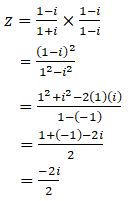= 0 – i

So now,

|Z| = √(x2 + y2)

= √(02 + (-1)2)

= √(0 + 1)

= √1

θ = tan-1 (|y|/ |x|)

= tan-1 (1/ 0)

= tan-1 ∞

Since x ≥ 0, y < 0complex number lies in 4th quadrant and the value of θ is -900≤θ≤00.

θ = -π/2

Z = 1 (cos (-π/2) + isin (-π/2))

= 1 (cos (π/2) – i sin(π/2))

Polar form of (1 – i)/ (1 + i) is 1 (cos (π/2) – i sin (π/2))

(v) 1/(1 + i)

Given: Z = 1 / (1 + i)

Let us multiply anddivide by (1 – i), we get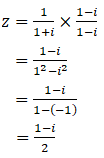So now,

|Z| = √(x2 + y2)

= √((1/2)2 + (-1/2)2)

= √(1/4 + 1/4)

= √(2/4)

= 1/√2

θ = tan-1 (|y|/ |x|)

= tan-1 ((1/2)/ (1/2))

= tan-1 1

Since x > 0, y <0 complex number lies in 4th quadrant and the value of θ is -900≤θ≤00.

θ = -π/4

Z = 1/√2 (cos (-π/4) + i sin (-π/4))

= 1/√2 (cos (π/4) – i sin (π/4))

Polar form of 1/(1 +i) is 1/√2 (cos (π/4) – i sin(π/4))

(vi) (1 + 2i) / (1 – 3i)

Given: Z = (1 + 2i) /(1 – 3i)

Let us multiply anddivide by (1 + 3i), we get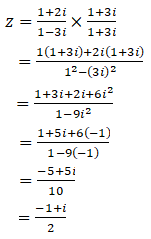So now,

|Z| = √(x2 + y2)

= √((-1/2)2 + (1/2)2)

= √(1/4 + 1/4)

= √(2/4)

= 1/√2

θ = tan-1 (|y|/ |x|)

= tan-1 ((1/2)/ (1/2))

= tan-1 1

Since x < 0, y >0 complex number lies in 2nd quadrant and the value of θ is 900≤θ≤1800.

θ = 3π/4

Z = 1/√2 (cos (3π/4) + i sin (3π/4))

Polar form of (1 +2i) / (1 – 3i) is 1/√2 (cos(3π/4) + i sin (3π/4))

(vii) sin 120o –i cos 120o

Given: Z = sin 120o –i cos 120o

= √3/2 – i (-1/2)

= √3/2 + i (1/2)

So now,

|Z| = √(x2 + y2)

= √((√3/2)2 + (1/2)2)

= √(3/4 + 1/4)

= √(4/4)

= √1

= 1

θ = tan-1 (|y|/ |x|)

= tan-1 ((1/2)/ (√3/2))

= tan-1 (1/√3)

Since x > 0, y >0 complex number lies in 1st quadrant and the value of θ is 00≤θ≤900.

θ = π/6

Z = 1 (cos (π/6) + isin (π/6))

Polar form of √3/2 + i (1/2) is 1 (cos (π/6) + i sin(π/6))

(viii) -16 / (1 + i√3)

Given: Z = -16 / (1 +i√3)

Let us multiply anddivide by (1 – i√3), we get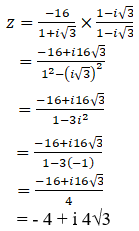So now,

|Z| = √(x2 + y2)

= √((-4)2 + (4√3)2)

= √(16 + 48)

= √(64)

= 8

θ = tan-1 (|y|/ |x|)

= tan-1 ((4√3) / 4)

= tan-1 (√3)

Since x < 0, y >0 complex number lies in 2nd quadrant and the value of θ is 900≤θ≤1800.

θ = 2π/3

Z = 8 (cos (2π/3) + isin (2π/3))

Polar form of -16 /(1 + i√3) is 8 (cos (2π/3) + i sin (2π/3))

Question 2 :

Write (i25)3 in polar form.

Given: Z = (i25)3

= i75

= i74. i

= (i2)37.i

= (-1)37. i

= (-1). i

= – i

= 0 – i

So now,

|Z| = √(x2 + y2)

= √(02 + (-1)2)

= √(0 + 1)

= √1

θ = tan-1 (|y|/ |x|)

= tan-1 (1/ 0)

= tan-1 ∞

Since x ≥ 0, y < 0complex number lies in 4th quadrant and the value of θ is -900≤θ≤00.

θ = -π/2

Z = 1 (cos (-π/2) + isin (-π/2))

= 1 (cos (π/2) – i sin(π/2))

Polar form of (i25)3 is1 (cos (π/2) – i sin (π/2))

Question 3 :

Express the following complex numbers in the form r (cos θ + i sinθ):

(i) 1 + i tan α

(ii) tan α – i

(iii) 1 – sin α + i cos α

(iv) (1 – i) / (cos π/3 + i sin π/3)

(i) 1 + i tan α

Given: Z = 1 + i tan α

We know that the polarform of a complex number Z = x + iy is given by Z = |Z| (cos θ + i sin θ)

Where,

|Z| = modulus of complexnumber = √(x2 +y2)

θ = arg (z) = argumentof complex number = tan-1 (|y| / |x|)

We also know that tanα is a periodic function with period π.

So α is lying in theinterval [0, π/2) (π/2, π].

Let us consider case1:

α [0, π/2)

So now,

|Z| = r = √(x2 + y2)

= √(12 + tan2 α)

= √( sec2 α)

= |sec α| since, sec αis positive in the interval [0, π/2)

θ = tan-1 (|y|/ |x|)

= tan-1 (tanα / 1)

= tan-1 (tanα)

= α since, tan α ispositive in the interval [0, π/2)

Polar form is Z = secα (cos α + i sin α)

Let us consider case2:

α (π/2, π]

So now,

|Z| = r = √(x2 + y2)

= √(12 + tan2 α)

= √( sec2 α)

= |sec α|

= – sec α since, sec αis negative in the interval (π/2, π]

θ = tan-1 (|y|/ |x|)

= tan-1 (tanα / 1)

= tan-1 (tanα)

= -π + α since, tan αis negative in the interval (π/2, π]

θ = -π + α [since, θlies in 4th quadrant]

Z = -sec α (cos (α –π) + i sin (α – π))

Polar form is Z =-sec α (cos (α – π) + i sin (α – π))

(ii) tan α – i

Given: Z = tan α – i

We know that the polarform of a complex number Z = x + iy is given by Z = |Z| (cos θ + i sin θ)

Where,

|Z| = modulus ofcomplex number = √(x2 +y2)

θ = arg (z) = argumentof complex number = tan-1 (|y| / |x|)

We also know that tanα is a periodic function with period π.

So α is lying in theinterval [0, π/2) (π/2, π].

Let us consider case1:

α [0, π/2)

So now,

|Z| = r = √(x2 + y2)

= √(tan2 α + 12)

= √( sec2 α)

= |sec α| since, sec αis positive in the interval [0, π/2)

= sec α

θ = tan-1 (|y|/ |x|)

= tan-1 (1/tanα)

= tan-1 (cotα) since, cot α is positive in the interval [0, π/2)

= α – π/2 [since, θlies in 4th quadrant]

Z = sec α (cos (α –π/2) + i sin (α – π/2))

Polar form is Z = secα (cos (α – π/2) + i sin (α – π/2))

Let us consider case2:

α (π/2, π]

So now,

|Z| = r = √(x2 + y2)

= √(tan2 α + 12)

= √( sec2 α)

= |sec α|

= – sec α since, sec αis negative in the interval (π/2, π]

θ = tan-1 (|y|/ |x|)

= tan-1 (1/tanα)

= tan-1 (cotα)

= π/2 + α since, cot αis negative in the interval (π/2, π]

θ = π/2 + α [since, θlies in 3th quadrant]

Z = -sec α (cos (π/2 +α) + i sin (π/2 + α))

Polar form is Z =-sec α (cos (π/2 + α) + i sin (π/2 + α))

(iii) 1 – sin α + icos α

Given: Z = 1 – sin α +i cos α

By using the formulas,

Sin2 θ+ cos2 θ = 1

Sin 2θ = 2 sin θ cos θ

Cos 2θ = cos2 θ– sin2 θ

So,

z= (sin2(α/2)+ cos2(α/2) – 2 sin(α/2) cos(α/2)) + i (cos2(α/2)– sin2(α/2))

= (cos(α/2) –sin(α/2))2 + i (cos2(α/2) – sin2(α/2))

We know that the polarform of a complex number Z = x + iy is given by Z = |Z| (cos θ + i sin θ)

Where,

|Z| = modulus ofcomplex number = √(x2 +y2)

θ = arg (z) = argumentof complex number = tan-1 (|y| / |x|)

Now,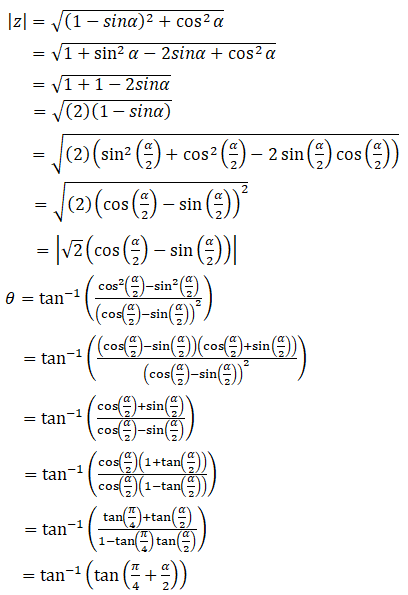We know that sine andcosine functions are periodic with period 2π

Here we have 3intervals:

0 ≤ α ≤ π/2

π/2 ≤ α ≤ 3π/2

3π/2 ≤ α ≤ 2π

Let us consider case1:

In the interval 0 ≤ α≤ π/2

Cos (α/2) > sin(α/2) and also 0 < π/4 + α/2 < π/2

So,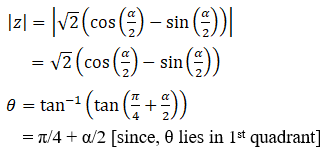Polar form is Z= √2 (cos (α/2) – sin(α/2)) (cos (π/4 + α/2) + i sin (π/4 + α/2))

Let us consider case2:

In the interval π/2 ≤α ≤ 3π/2

Cos (α/2) < sin(α/2) and also π/2 < π/4 + α/2 < π

So,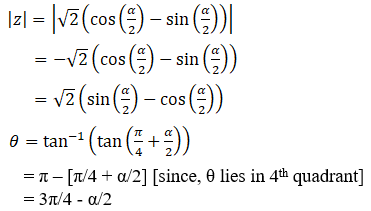Since, (1 – sin α)> 0 and cos α < 0 [Z lies in 4th quadrant]

= α/2 – 3π/4

Polar form is Z = –√2 (cos (α/2) – sin (α/2)) (cos (α/2 –3π/4) + i sin (α/2 – 3π/4))

Let us consider case3:

In the interval 3π/2 ≤α ≤ 2π

Cos (α/2) < sin(α/2) and also π < π/4 + α/2 < 5π/4

So,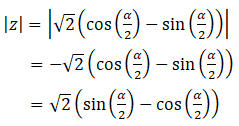θ = tan-1 (tan(π/4 + α/2))

= π – (π/4 + α/2)[since, θ lies in 1st quadrant and tan’s period is π]

= α/2 – 3π/4

Polar form is Z = –√2 (cos (α/2) – sin (α/2)) (cos (α/2 –3π/4) + i sin (α/2 – 3π/4))

(iv) (1 – i) / (cos π/3 + isin π/3)

Given: Z = (1 – i) /(cos π/3 + i sin π/3)

Let us multiply anddivide by (1 – i√3), we get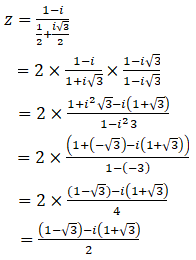We know that the polarform of a complex number Z = x + iy is given by Z = |Z| (cos θ + i sin θ)

Where,

|Z| = modulus ofcomplex number = √(x2 +y2)

θ = arg (z) = argumentof complex number = tan-1 (|y| / |x|)

Now,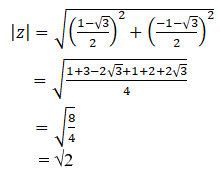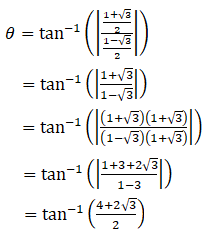Since x < 0, y <0 complex number lies in 3rd quadrant and the value of θ is 1800≤θ≤-900.

= tan-1 (2+ √3)

= -7π/12

Z = √2 (cos (-7π/12) + i sin (-7π/12))

= √2 (cos (7π/12) – i sin (7π/12))

Polar form of (1 – i)/ (cos π/3 + i sin π/3) is √2(cos (7π/12) – i sin (7π/12))

Question 4 :

If z1 and z2 are two complex number suchthat |z1| = |z2| and arg (z1) + arg (z2)= π, then show that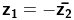|z1| = |z2|and arg (z1) + arg (z2) = π

Let us assume arg (z1)= θ

arg (z2) =π – θ

We know that in thepolar form, z = |z| (cos θ + i sin θ)

z=|z1| (cos θ + i sin θ) …………. (i)

z=|z2| (cos (π – θ) + i sin (π – θ))

= |z2|(-cos θ + i sin θ)

= – |z2|(cos θ – i sin θ)

Now let us find theconjugate of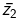= – |z2|(cos θ + i sin θ) …… (ii) (since, |Z2¯¯¯¯¯¯|=|Z2|)

Now,

z1 /= [|z1| (cos θ + i sinθ)] / [-|z2| (cos θ + i sin θ)]

= – |z1| /|z2| [since, |z1| = |z2|]

= -1

When we cross multiplywe get,

z1 = –Hence proved.

Question 5 :

If z1, z2 and z3, z4 aretwo pairs of conjugate complex numbers, prove that arg (z1/z4)+ arg (z2/z3) = 0

Given: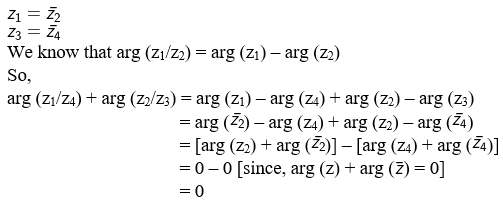Hence proved.

Question 6 :

Express sin π/5 + i (1 – cos π/5) in polar form.

Given:

Z = sin π/5 + i (1 –cos π/5)

By using the formula,

sin 2θ = 2 sin θ cos θ

1- cos 2θ = 2 sin2 θ

So,

Z = 2 sin π/10 cosπ/10 + i (2 sin2 π/10)

= 2 sin π/10 (cos π/10+ i sin π/10)

The polar form of sinπ/5 + i (1 – cos π/5) is 2 sin π/10 (cos π/10 + i sin π/10)

Todays Deals### RD Chapter 13- Complex Numbers Ex-13.4 Contributorskrishan

Name:
Email:

# Latest News# 9000 interview questions in different categories